# Test Prep Plan - Take a practice test

Take this practice test to check your existing knowledge of the course material. We'll review your answers and create a Test Prep Plan for you based on your results.
How Test Prep Plans work
1
2Based on your results, we'll create a customized Test Prep Plan just for you!
3Study smarter
Study more effectively: skip concepts you already know and focus on what you still need to learn.

# FTCE Math: Rate of Change Chapter Exam

Exam Instructions:

Choose your answers to the questions and click 'Next' to see the next set of questions. You can skip questions if you would like and come back to them later with the yellow "Go To First Skipped Question" button. When you have completed the practice exam, a green submit button will appear. Click it to see your results. Good luck!

### Page 1

#### Question 1 1. What is the derivative of the following?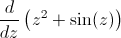#### Question 2 2. What is the rate of change at the point B in the graph below (for y in relation to x)?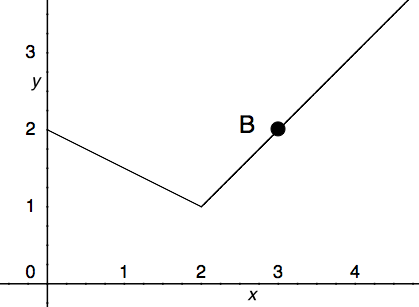#### Question 4 4. What is the average rate of change for the interval with endpoints A and B?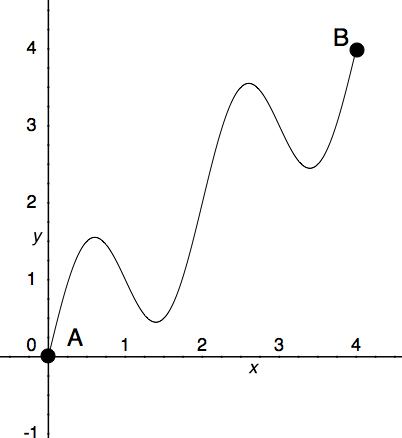#### Question 5 5. What is the rate of change at the point A in the graph below (for y in relation to x)?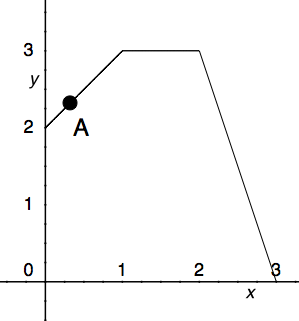### Page 2

#### Question 6 6. According to the mean value theorem, at how many points will the instantaneous rate of change equal the average rate of change between x = 1 and x = 3 on the following graph?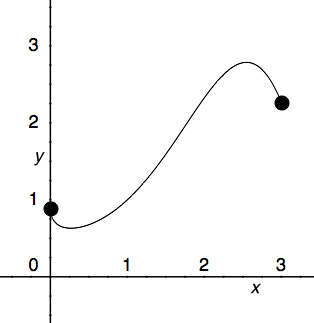#### Question 7 7. What is the approximate instantaneous rate of change at point C?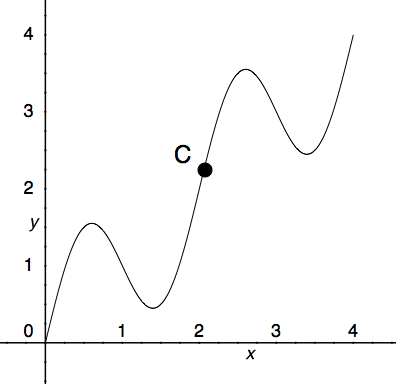#### Question 8 8. Which is greater, the derivative at a or the derivative at b?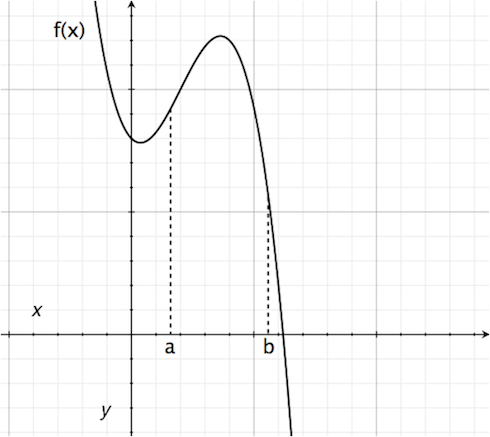#### Question 9 9. At how many points on the graph below is the derivative of f(x)=0?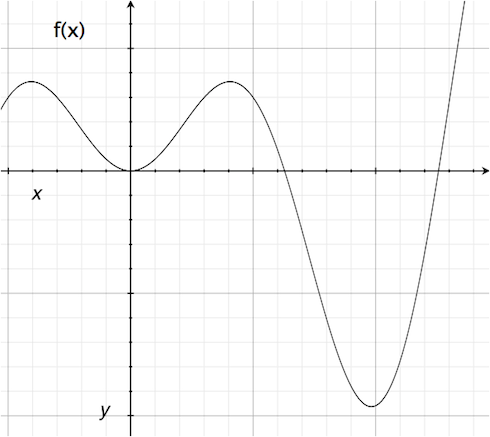### Page 3

#### Question 11 11. What is the value of f(x) at the red circle?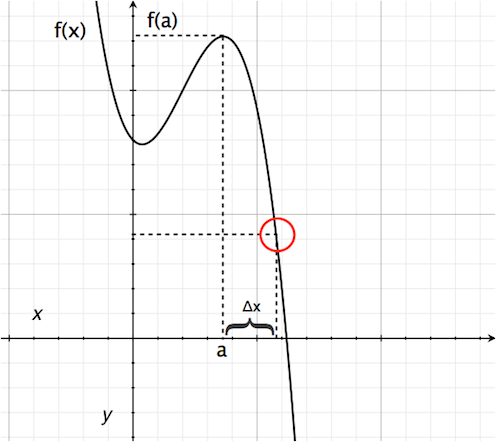#### Question 13 13. What is the rate of change at the point E in the graph below (for y in relation to x)?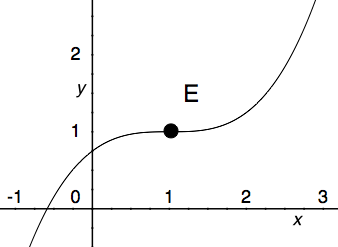#### Question 15 15. Where is f(x) differentiable?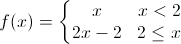### Page 4

#### Question 16 16. What is the rate of change at the point B in the graph below (for y in relation to x)? (In other words, what is the slope of the line from x = 2 to x = 3?)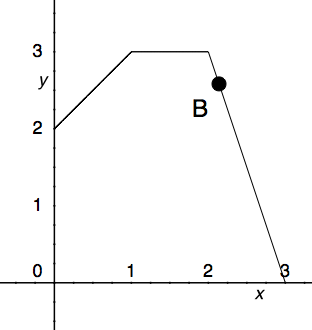#### Question 18 18. Where is f(x) differentiable?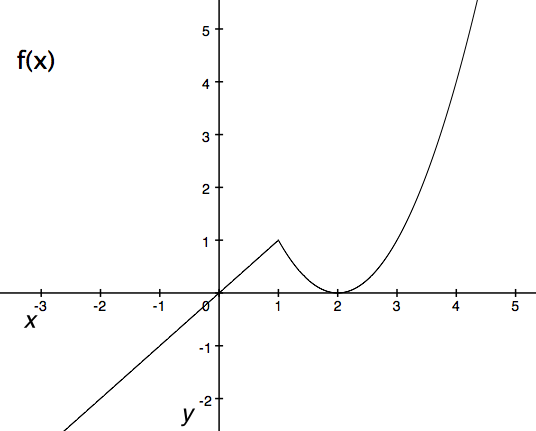#### Question 19 19. Where is f(x) differentiable?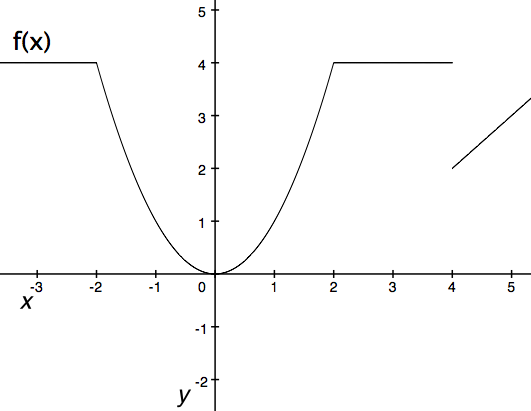### Page 5

#### Question 21 21. What is the rate of change at the point A in the graph below (for y in relation to x)?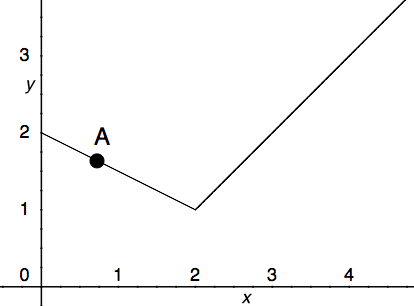#### Question 23 23. Where is f(x) differentiable?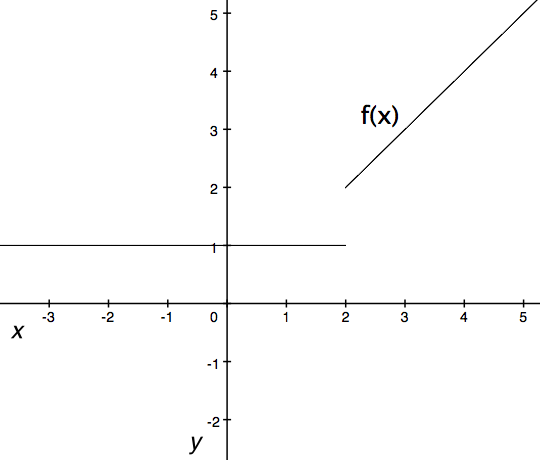#### Question 24 24. Consider the graph and question below..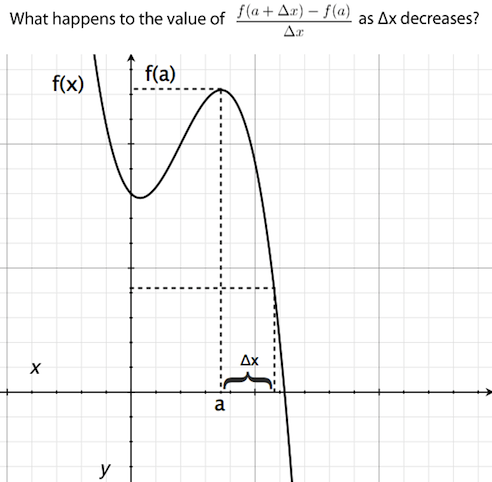#### Question 25 25. Where t= time, what is the derivative of f(t)?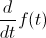### Page 6

#### Question 27 27. Which point on the following graph satisfies the mean value theorem between x = 0 and x = 3?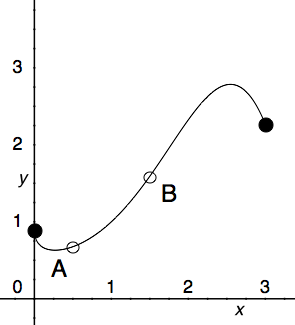#### Question 28 28. The derivative of f(x) is equal to 0 at how many points on the graph below?#### Question 29 29. Where is y differentiable?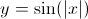#### FTCE Math: Rate of Change Chapter Exam Instructions

Choose your answers to the questions and click 'Next' to see the next set of questions. You can skip questions if you would like and come back to them later with the yellow "Go To First Skipped Question" button. When you have completed the practice exam, a green submit button will appear. Click it to see your results. Good luck!

Support